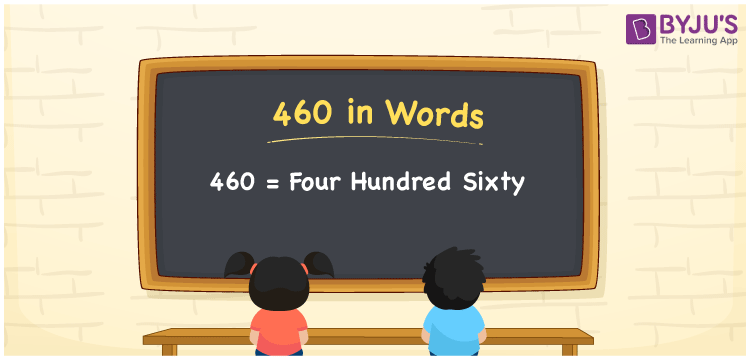# 460 in Words

The number 460 in English is expressed as Four Hundred Sixty. For instance, Rohan purchased a butterscotch cake worth Rs. 460 for his friend, then you can say, “Rohan purchased a butterscotch cake worth Rupees Four Hundred Sixty for his friend”. We can convert the number to words by using place values such as ones, tens, hundreds, and so on. 460 is a cardinal number as it depicts a certain amount. In this article, let us study the spelling of 460 in English and also how to write the numerical name of 460 using a place value chart.

 460 in Words Four Hundred Sixty Four Hundred Sixty in numerical form 460

## 460 in English Words

English is the most commonly used language in education. In Maths, generally, we write numbers in words using the English alphabet. Thus, we can write the number 460 in words as Four Hundred Sixty.## How to Write 460 in Words?

It is vital to find the place value of each digit in the given number while converting in word form. 460 is a three-digit number. Hence, we need a table of 3 columns to write its numerical name. The below table shows the place value chart for the number 460.

 Hundreds Tens Ones 4 6 0

Hence, we can write the expanded form as:

4 x Hundred + 6 x Ten + 0 x One

= 4 x 100 + 6 x 10 + 0 x 1

= 400 + 60 + 0

= 400 + 60

= 460

= Four Hundred Sixty

Therefore, 460 in words is written as Four Hundred Sixty

Interesting way of writing 460 in words

4 = Four

46 = Forty-Six

460 = Four Hundred Sixty

Thus, the word form of the number 460 is Four Hundred Sixty

460 is a natural number that is the successor of 459 and the predecessor of 461

• 460 in words – Four Hundred Sixty
• Is 460 an odd number? – No
• Is 460 an even number? – Yes
• Is 460 a perfect square number? – No
• Is 460 a perfect cube number? – No
• Is 460 a prime number? – No
• Is 460 a composite number? – Yes

## Frequently Asked Questions on 460 in Words

### Write 460 in words.

460 in words is written as Four Hundred Sixty.

### Simplify 500 – 40, and express in words.

Simplifying 500 – 40, we get 460. The number 460 in words is Four Hundred Sixty.

### Is 460 an even number?

Yes, the number 460 is an even number.